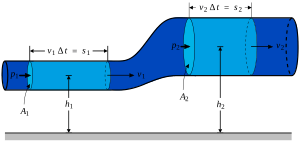# Fluid dynamics facts for kids

Kids Encyclopedia Facts

MechanicsFluid Dynamics talks about how fluids (liquids and gases) work. It is one of the oldest parts of the study of Physics, and is studied by physicists, mathematicians, and engineers. Mathematics can describe how fluids move using mathematical formulas called equations. The fluid dynamics of gases are called aerodynamics.

Understanding how fluids behave helps us understand things like flight or ocean currents. For example, fluid dynamics can be used to understand weather, because clouds and air are both fluids. Fluid dynamics can also be used to understand how aeroplanes fly through the air or how ships and submarines move through water.

Computer programs can use the mathematical equations of fluid dynamics to model and predict the actions of moving fluids. Computers have helped us understand fluid dynamics very much, and some people study how to model or simulate fluids only with a computer. Studying how fluid dynamics can be done with computers is called computational fluid dynamics (or CFD for short).

## Important equations in fluid dynamics

The mathematical equations that govern fluid flow are simple to think about but very hard to solve. In most real life cases there is no way to get an solution that can be written down and a computer must be used to calculate the answer instead. There are three fundamental equations based on three principles.

Conservation of mass: mass is neither created nor destroyed, it simply moves from one place to another. This gives the mass conservation equation. Sometimes this may not apply such as a flow involving a chemical reaction.

Conservation of energy: this is the first law of thermodynamics, energy is never created or destroyed, it just changes form (i.e. kinetic energy into potential energy) or moves around.

Conservation of momentum: this is Newton's Second Law and it states that Force = rate of change of momentum. Momentum is mass times velocity. The momentum equations are the equations that make it hard to solve problems in fluid dynamics. There are a number of different versions that include a number of different effects. The Navier-Stokes equations are momentum equations, and the Euler equations are the Navier-Stokes equations but with viscosity not included. There is one momentum equation in a 1D problem and three, one in each space direction, in 3D.

To solve the equations more information is often needed in the form of an equation of state. This relates thermodynamic properties (usually pressure and temperature) to each other for a specific type of fluid. An example is the "Ideal Gas" equation of state that relates pressure, temperature and density and works well for gases under normal pressures (like air at atmospheric pressure).

• Poiseuille's Equation
• Bernoulli's Theorem
• Navier-Stokes Equations

## Images for kidsFluid dynamics Facts for Kids. Kiddle Encyclopedia.